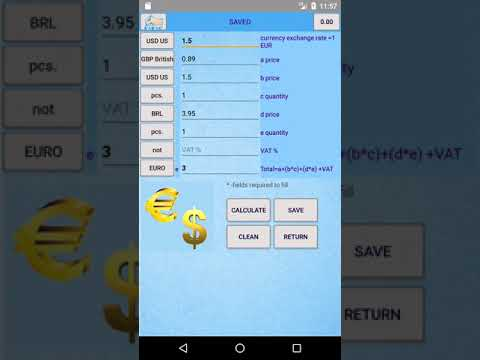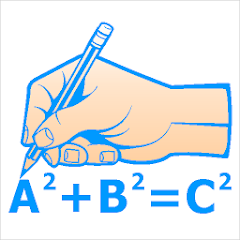# Formulas Calc Unit Converter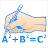50K+Everyone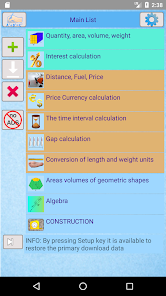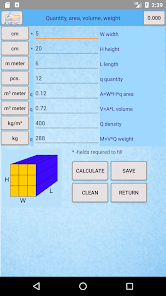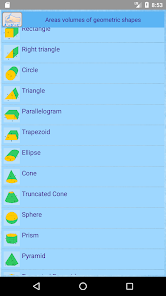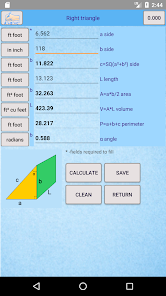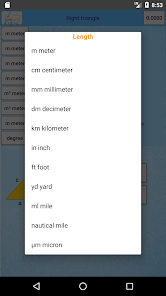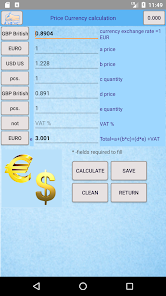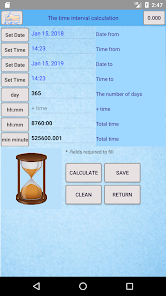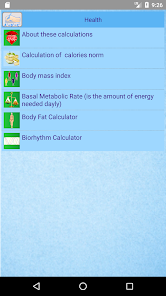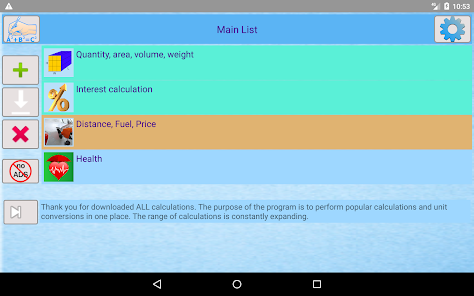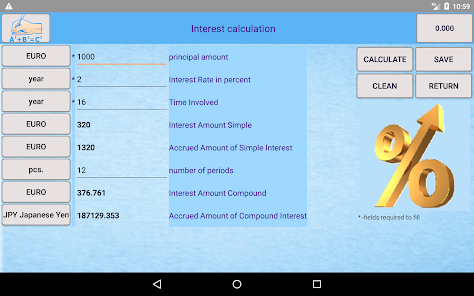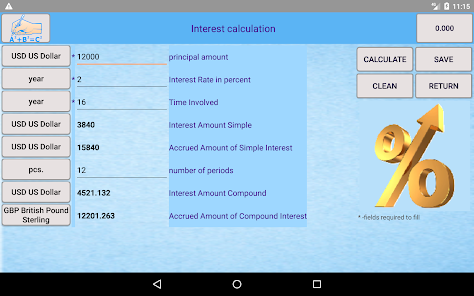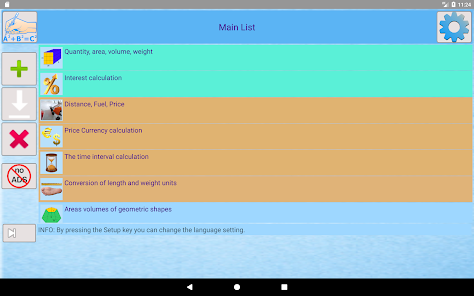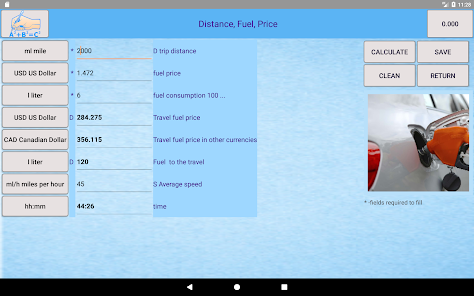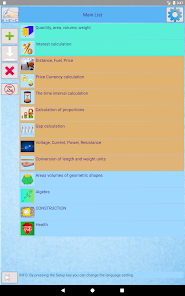This app performs popular calculations and unit conversions. The range of calculations is constantly expanding.

The application is defined by dynamic change of units. You can fill in one units of measurments and get the results in another selected units. There are about 300 units possible. Along with more complex calculations you will find a drawing.

Calculation results can be saved to make a template for the next calculation. Minimum data should be entered for the next calculation.Calculations in selected units needed for often the necessary calculations.

Currently there are calculations from the following areas:
The most popular geometrics shapes, diagonal, perimeter, area, volume formulas and calculations.
Calculation for CONSTRUCTION in selected measurment units.
Other calculations: interest, time interval, proportions, gap, mass, ...
Updated on
Oct 27, 2020

## Data safety